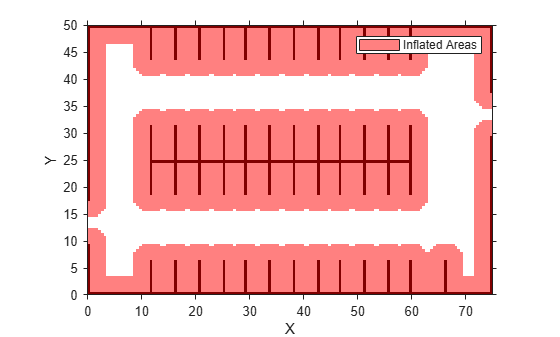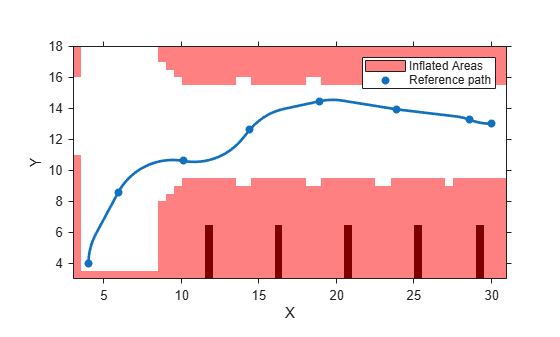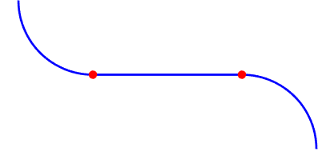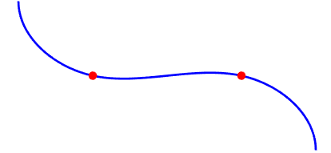Documentation

### This is machine translation

Mouseover text to see original. Click the button below to return to the English version of the page.

# smoothPathSpline

Smooth vehicle path using cubic spline interpolation

## Syntax

``[poses,directions] = smoothPathSpline(refPoses,refDirections,numSmoothPoses)``
``[poses,directions] = smoothPathSpline(refPoses,refDirections,numSmoothPoses,minSeparation)``
``[___,cumLength,curvatures] = smoothPathSpline(___)``

## Description

example

````[poses,directions] = smoothPathSpline(refPoses,refDirections,numSmoothPoses)` generates a smooth vehicle path, consisting of `numSmoothPoses` discretized poses, by fitting the input reference path poses to a cubic spline. Given the input reference path directions, `smoothPathSpline` also returns the directions that correspond to each pose.Use this function to convert a C1-continuous vehicle path to a C2-continuous path. C1-continuous paths include the `driving.DubinsPathSegment` or `driving.ReedsSheppPathSegment` paths that you can plan using a `pathPlannerRRT` object. For more details on these path types, see C1-Continuous and C2-Continuous Paths.You can use the returned poses and directions with a vehicle controller, such as the `lateralControllerStanley` function.```
````[poses,directions] = smoothPathSpline(refPoses,refDirections,numSmoothPoses,minSeparation)` specifies a minimum separation threshold between poses. If the distance between two poses is smaller than `minSeparation`, the function uses only one of the poses for interpolation.```
````[___,cumLength,curvatures] = smoothPathSpline(___)` also returns the cumulative path length and signed path curvature at each returned pose, using any of the previous syntaxes. Use these values to generate a speed profile along the path. ```

## Examples

collapse all

Smooth a path that was planned by an RRT* path planner.

Load and plot a costmap of a parking lot.

```data = load('parkingLotCostmap.mat'); costmap = data.parkingLotCostmap; plot(costmap)```Define start and goal poses for the vehicle as [x, y, Θ] vectors. World units for the (x,y) locations are in meters. World units for the Θ orientation angles are in degrees.

```startPose = [4,4,90]; % [meters, meters, degrees] goalPose = [30,13,0];```

Use a `pathPlannerRRT` object to plan a path from the start pose to the goal pose.

```planner = pathPlannerRRT(costmap); refPath = plan(planner,startPose,goalPose);```

Plot and zoom in on the planned path. The path is composed of a sequence of Dubins curves. These curves include abrupt changes in curvature that are not suitable for driving with passengers.

```hold on plot(refPath,'Vehicle','off','DisplayName','Reference path') xlim([3 31]) ylim([3 18])```Interpolate the transition poses of the path. Use these poses as the reference poses for interpolating the smooth path. Also return the motion directions at each pose.

`[refPoses,refDirections] = interpolate(refPath);`

Specify the number of poses to return in the smooth path. Return poses spaced about 0.1 meters apart, along the entire length of the path.

```approxSeparation = 0.1; % meters numSmoothPoses = round(refPath.Length / approxSeparation);```

Generate the smooth path by fitting a cubic spline to the reference poses. `smoothPathSpline` returns the specified number of discretized poses along the smooth path.

`[poses,directions] = smoothPathSpline(refPoses,refDirections,numSmoothPoses);`

Plot the smooth path. The more abrupt changes in curvature that were present in the reference path are now smoothed out.

```plot(poses(:,1),poses(:,2),'LineWidth',2,'DisplayName','Smooth path') hold off```## Input Arguments

collapse all

Reference poses of the vehicle along the path, specified as an M-by-3 matrix of [x, y, Θ] vectors, where M is the number of poses.

x and y specify the location of the vehicle in meters. Θ specifies the orientation angle of the vehicle in degrees.

Data Types: `single` | `double`

Reference directions of the vehicle along the path, specified as an M-by-1 column vector of 1s (forward motion) and –1s (reverse motion). M is the number of reference directions. Each element of `refDirections` corresponds to a pose in the `refPoses` input argument.

Data Types: `single` | `double`

Number of smooth poses to return in the `poses` output argument, specified as a positive integer. To increase the granularity of the returned poses, increase `numSmoothPoses`.

Minimum separation between poses, in meters, specified as a positive real scalar. If the Euclidean (x, y) distance between two poses is less than this value, then the function uses only one of these poses for interpolation.

## Output Arguments

collapse all

Discretized poses of the smoothed path, returned as a `numSmoothPoses`-by-3 matrix of [x, y, Θ] vectors.

x and y specify the location of the vehicle in meters. Θ specifies the orientation angle of the vehicle in degrees.

The values in `poses` are of the same data type as the values in the `refPoses` input argument.

Motion directions at each output pose in `poses`, returned as a `numSmoothPoses`-by-1 column vector of 1s (forward motion) and –1s (reverse motion).

The values in `directions` are of the same data type as the values in the `refDirections` input argument.

Cumulative path lengths at each output pose in `poses`, returned as a `numSmoothPoses`-by-1 real-valued column vector. Units are in meters.

You can use the `cumLength` and `curvatures` outputs to generate a speed profile of the vehicle along the smooth path. For more details, see the Automated Parking Valet example.

Signed path curvatures at each output pose in `poses`, returned as a `numSmoothPoses`-by-1 real-valued column vector. Units are in radians per meter.

You can use the `curvatures` and `cumLength` outputs to generate a speed profile of the vehicle along the smooth path. For more details, see the Automated Parking Valet example.

collapse all

### C1-Continuous and C2-Continuous Paths

A path is C1-continuous if its derivative exists and is continuous. Paths that are only C1-continuous have discontinuities in their curvature. For example, a path composed of Dubins or Reeds-Sheep path segments has discontinuities in curvature at the points where the segments join. These discontinuities result in changes in direction that are not smooth enough for driving with passengers.A path is also C2-continuous if its second derivative exists and is continuous. C2-continuous paths have continuous curvature and are smooth enough for driving with passengers.## Algorithms

• The path-smoothing algorithm interpolates a parametric cubic spline that passes through all input reference pose points. The parameter of the spline is the cumulative chord length at these points. 

• The tangent direction of the smoothed output path approximately matches the orientation angle of the vehicle at the starting and goal poses.

 Floater, Michael S. "On the Deviation of a Parametric Cubic Spline Interpolant from Its Data Polygon." Computer Aided Geometric Design. Vol. 25, Number 3, 2008, pp. 148–156.

 Lepetic, Marko, Gregor Klancar, Igor Skrjanc, Drago Matko, and Bostjan Potocnik. "Time Optimal Path Planning Considering Acceleration Limits." Robotics and Autonomous Systems. Vol. 45, Numbers 3–4, 2003, pp. 199–210.